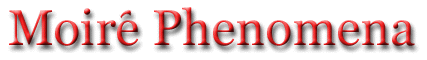Moire Radial Multi-Color Rotation, Translation Two animated thick radial patterns - illustrates a rotating dipole magnetic field (as in a pulsar). Two radial patterns with equal spacing Two radial patterns with equal spacing - ver. 2 (more dense) Two radial patterns with equal spacing in blue Two radial patterns in blue with only center with translation & rotation Two thick blue radial patterns with translation & rotation Two linear gratings (vertical, line:space = 1:2) - illustrates superluminal phase velocity. Two linear gratings - (vertical, line:space = 1:3) - illustrates superluminal phase velocity. Two linear gratings (offset, line:space = 1:4): - illustrates superluminal phase velocity. Two thick linear gratings (offset, line:space = 1:2) - illustrates superluminal phase velocity. Two animated variable-spacing linear gratings - illustrates beat phenomena Two overlapping zone plates Two overlapping thick concentric ring patterns Two concentric ring patterns with equal spacing Two different concentric ring patterns Spiral and concentric rings